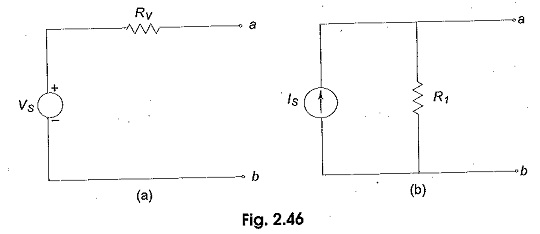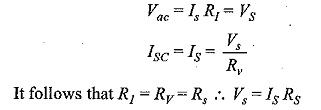## Source Transformation Technique:

Source Transformation Technique – In solving networks to find solutions one may have to deal with energy sources. It has already been discussed before that basically, energy Source Transformation are either voltage sources or current sources. Sometimes it is necessary to convert a voltage source to a current source and vice-versa. Any practical voltage source consists of an ideal voltage source in series with an internal resistance. Similarly, a practical current source consists of an ideal current source in parallel with an internal resistance as shown in Fig. 2.46. Rv and Ri represent the internal resistances of the voltage source Vs, and current source Is respectively.

Any Source Transformation, be it a current source or a voltage source, drives current through its load resistance, and the magnitude of the current depends on the value of the load resistance. Figure 2.47 represents a practical voltage source and a practical current source connected to the same load resistance RL.From Fig. 2.47 (a), the load voltage can be calculated by using Kirchhoff’s voltage law asThe above two Source Transformation are said to be equal, if they produce equal amounts of current and voltage when they are connected to identical load resistances. Therefore, by equating the open circuit voltages and short circuit currents of the above two sources we obtainwhere Rs is the internal resistance of the voltage or current source. Therefore, any practical voltage source, having an ideal voltage Vs and internal series resistance Rs can be replaced by a current source Vs/Rs in parallel with an internal resistance Rs. The reverse transformation is also possible. Thus, a practical current source in parallel with an internal resistance Rs can be replaced by a voltage source Vs = Is Rs in series with an internal resistance Rs.

Scroll to Top